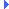FD.io VPP  v20.05-21-gb1500e9ff Vector Packet Processing
random.h File Reference

Linear Congruential Random Number Generator. More...Include dependency graph for random.h:This graph shows which files directly or indirectly include this file:

Go to the source code of this file.

## Functions

static u32 random_u32 (u32 *seed)
32-bit random number generator More...

int test_random_main (unformat_input_t *input)

static u32 random_u32_max (void)
Maximum value returned by random_u32() More...

static uword random_default_seed (void)
Default random seed (unix/linux user-mode) More...

static u64 random_u64 (u64 *seed)
64-bit random number generator Again, constants courtesy of Donald Knuth. More...

static uword random_uword (u32 *seed)
machine word size random number generator More...

static f64 random_f64 (u32 *seed)
Generate f64 random number in the interval [0,1]. More...

static u8random_string (u32 *seed, uword len)
Generate random character vector. More...

f64 clib_chisquare (u64 *values)
Compute the X2 test statistic for a vector of counts. More...

## Detailed Description

Linear Congruential Random Number Generator.

This specific random number generator is described in "Numerical Recipes in C", 2nd edition, page 284. If you need random numbers with really excellent statistics, take a look at Chapter 7...

By definition, a linear congruential random number generator is of the form: rand[i+1] = a*rand[i] + c (mod m) for specific values of (a,c,m).

In this case, choose m = 2**32 and use the low-order 32-bits of the 64-bit product a*N[i]. Knuth suggests the use of a=1664525, H.W. Lewis has tested C=1013904223 extensively. This routine is reputedly as good as any 32-bit LCRN, and costs only a single multiply-add.

Several variants: 32/64-bit, machine word width, f64 on the closed interval [0,1].

Definition in file random.h.

## ◆ clib_chisquare()

 f64 clib_chisquare ( u64 * values )

Compute the X2 test statistic for a vector of counts.

Each value element corresponds to a histogram bucket.

Typical use-case: test the hypothesis that a set of octets are uniformly distributed (aka random).

In a 1-dimensional use-case, the result should be compared with the critical value from chi square tables with vec_len(values) - 1 degrees of freedom.

Parameters
 [in] values vector of histogram bucket values
Returns
d - Pearson's X2 test statistic

Definition at line 65 of file random.c.Here is the caller graph for this function:

## ◆ random_default_seed()

 static uword random_default_seed ( void )
inlinestatic

Default random seed (unix/linux user-mode)

Definition at line 91 of file random.h.Here is the caller graph for this function:

## ◆ random_f64()

 static f64 random_f64 ( u32 * seed )
inlinestatic

Generate f64 random number in the interval [0,1].

Definition at line 145 of file random.h.Here is the call graph for this function:Here is the caller graph for this function:

## ◆ random_string()

 static u8* random_string ( u32 * seed, uword len )
inlinestatic

Generate random character vector.

From the alphabet a-z, lower case. Returns a vector of the supplied length which is NOT guaranteed to be NULL-terminated. FIXME?

Definition at line 157 of file random.h.Here is the call graph for this function:

## ◆ random_u32()

 static u32 random_u32 ( u32 * seed )
inlinestatic

32-bit random number generator

Definition at line 69 of file random.h.Here is the call graph for this function:Here is the caller graph for this function:

## ◆ random_u32_max()

 static u32 random_u32_max ( void )
inlinestatic

Maximum value returned by random_u32()

Definition at line 80 of file random.h.Here is the caller graph for this function:

## ◆ random_u64()

 static u64 random_u64 ( u64 * seed )
inlinestatic

64-bit random number generator Again, constants courtesy of Donald Knuth.

Definition at line 126 of file random.h.Here is the caller graph for this function:

## ◆ random_uword()

 static uword random_uword ( u32 * seed )
inlinestatic

machine word size random number generator

Definition at line 135 of file random.h.Here is the call graph for this function:

## ◆ test_random_main()

 int test_random_main ( unformat_input_t * input )Here is the caller graph for this function: